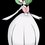# Help in these Problems

Problems:

1. If x + y + xy = 1, where x and y are non-zero real numbers, what is xy + 1/xy - y/x - x/y? (The answer is 4 but needing simple algebraic manipulation.)

2. The quartic polynomial P(x) satisfies P(1) = 0 and attains its maximum value of 3 at both x = 2 and x = 3. Compute P(5). More appreciated if the solution does not require calculus at least.

3. Let S(X) be the sum of elements of a nonempty finite set X, where X is a set of numbers. Calculate the sum of all numbers S(X) where X ranges over all nonempty subsets of the set {1, 2, 3, ..., 16}. Please show quick method.

-From PMONote by John Ashley Capellan
6 years, 8 months ago

This discussion board is a place to discuss our Daily Challenges and the math and science related to those challenges. Explanations are more than just a solution — they should explain the steps and thinking strategies that you used to obtain the solution. Comments should further the discussion of math and science.

When posting on Brilliant:

• Use the emojis to react to an explanation, whether you're congratulating a job well done , or just really confused .
• Ask specific questions about the challenge or the steps in somebody's explanation. Well-posed questions can add a lot to the discussion, but posting "I don't understand!" doesn't help anyone.
• Try to contribute something new to the discussion, whether it is an extension, generalization or other idea related to the challenge.

MarkdownAppears as
*italics* or _italics_ italics
**bold** or __bold__ bold
- bulleted- list
• bulleted
• list
1. numbered2. list
1. numbered
2. list
Note: you must add a full line of space before and after lists for them to show up correctly
paragraph 1paragraph 2

paragraph 1

paragraph 2

[example link](https://brilliant.org)example link
> This is a quote
This is a quote
    # I indented these lines
# 4 spaces, and now they show
# up as a code block.

print "hello world"
# I indented these lines
# 4 spaces, and now they show
# up as a code block.

print "hello world"
MathAppears as
Remember to wrap math in $$ ... $$ or $ ... $ to ensure proper formatting.
2 \times 3 $2 \times 3$
2^{34} $2^{34}$
a_{i-1} $a_{i-1}$
\frac{2}{3} $\frac{2}{3}$
\sqrt{2} $\sqrt{2}$
\sum_{i=1}^3 $\sum_{i=1}^3$
\sin \theta $\sin \theta$
\boxed{123} $\boxed{123}$

Sort by:

1. Put $y = \tfrac{1-x}{1+x}$. Then $\begin{array}{rcl} xy + \frac{1}{xy} & = & \frac{x(1-x)}{1+x} + \frac{1+x}{x(1-x)} \; = \; \frac{x^2(1-x)^2 + (1+x)^2}{x(1-x^2)} \\ \frac{x}{y} + \frac{y}{x} & = & \frac{x(1+x)}{1-x} + \frac{1-x}{x(1+x)} \; = \; \frac{x^2(1+x)^2 + (1-x)^2}{x(1-x^2)} \end{array}$ and so $\begin{array}{rcl} xy + \frac{1}{xy} - \frac{x}{y} - \frac{y}{x} & = & \frac{(1+x)^2(1-x^2) - (1-x)^2(1-x^2)}{x(1-x^2)} \\ & = & \frac{(1+x)^2 - (1-x)^2}{x} \; = \; 4 \end{array}$

2. The polynomial is $P(x) = 3 - \tfrac34(x-2)^2(x-3)^2$, and so $P(5) = -24$.

3. For simplicity, let $S(\varnothing) = 0$, so that $X$ can range over all subsets of $\{1,2,\ldots,n\}$. Each number $1 \le j \le n$ occurs in precisely half of the $2^n$ subsets of $\{1,2,\ldots,n\}$, and so contributes a total of $j \times 2^{n-1}$ to the total sum $S_\mathsf{total} \; = \; \sum_{X \subseteq\{1,2,\ldots,n\}} S(X)$ Thus $S_\mathsf{tot} \; = \; 2^{n-1}\sum_{j=1}^n j \; = \; n(n+1)2^{n-2}$

- 6 years, 8 months ago

Hello.. I just noticed that For x = 1, the value is not 0... contradicting the given...

- 6 years, 8 months ago

So, OK, if $x=1$ then $y=0$. This just means that for $x$ and $y$ to be nonzero and satisfy the identity $x+y+xy=1$, they also have to be not equal to $1$. That just means that there is an additional "hidden" restriction on the possible values of $x$ and $y$, but nothing more. If you think about it, $x$ and $y$ cannot be equal to $-1$, either.

- 6 years, 8 months ago

Oh.. sorry.. I was talking about the second problem, not the first... Sorry for not mentioning the number...

- 6 years, 8 months ago

Good point. I have corrected the solution, which needed $\tfrac34$ instead of $\tfrac14$.

- 6 years, 8 months ago